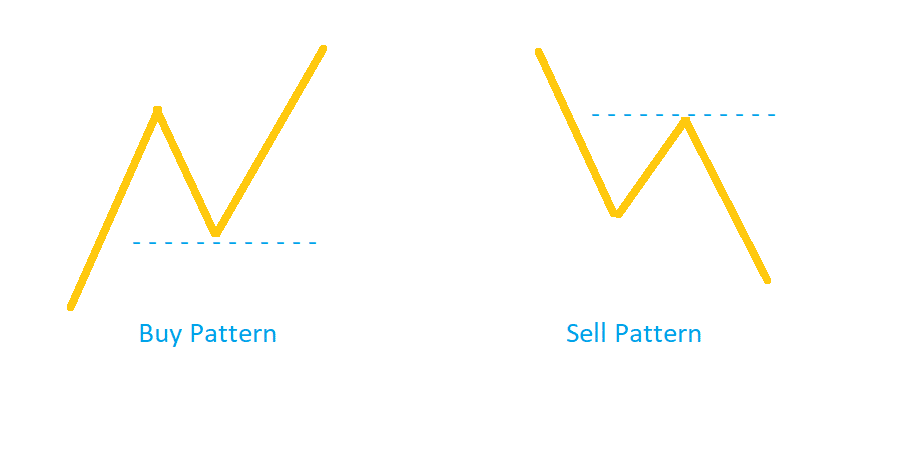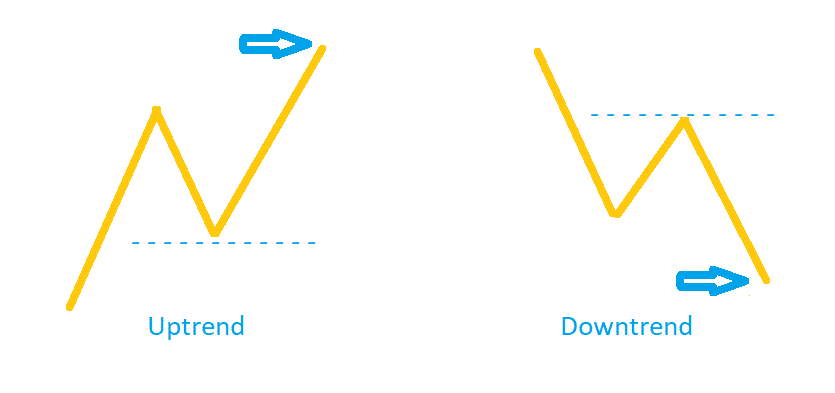# Fibonacci Retracement and Extension

Not only is the Fibonacci retracement level the trusted tool of many millions of traders out there, there's also some crazy history and mad secrets and patterns that repeat throughout nature. It's no different when trading, and you can exploit this to supercharge your trades if you know what you're doing.

### Fibonacci Sequence: Ground Zero

The Fibonacci sequence starts at 1, and each number thereon is an addition of the number itself and the preceding number.

1              1              2              3              5              8              13           21           34           55           89           … to ∞

Several noteworthy patterns emerge in the Fibonacci sequence:

• Dividing any number by the next number in the series gives 0.618
• Diving any number by the number 2 places after it in the series gives 0.382
• Dividing any number by the number 3 places after it in the series gives 0.236

These numbers are popularly referred to as Fibonacci ratios. If you divide any 2 Fibonacci ratios, you will get either X.618 or X.382.

Similarly, dividing a number with a number preceding it in the series always gives us 1.618; this ratio is often referred to as the Golden Ratio or ‘Phi.’

If this is your first time looking at the Fibonacci sequence, note that the relationship between the numbers in the sequence emerges as you tend towards infinity, and might not be as strong for the first few numbers.

### Fibonacci Retracement Levels

The most commonly used Fibonacci retracement levels technical analysts use are 23.6%, 38.2%, 50%, 61.8%, and 78.6%. All but one (50%) of these numbers are pulled out of the Fibonacci sequence. 50% is still used widely because some technical analysts contend that a drawdown tends to retrace close to half its preceding advance.

Most traders ignore the decimal on these numbers since these are supposed to work as estimates anyway. Fibonacci retracement levels are not a silver bullet, but they are an extremely useful tool for forex traders.

For instance, let’s say you are taking a bet on the GBP/JPY pair. It moves about 50 pips upward to 150.50 from 150. In this case, a 23.6% retracement would mean the quote will pull back 12 pips to 150.38.

Fibonacci retracements work best on high-volume currency pairs. A low-volume pair is influenced by few individuals, which means it is more prone to irregular movements that will not align with the Fibonacci retracement levels.

### Using Fibonacci Retracement Levels

Fibonacci retracement levels help traders establish a support and resistance level which suggests a point of a possible reversal in the trend’s direction. In turn, this information helps traders identify a good time to open a position.

The retracement levels look back at the previous market movements. After a significant spike in the price of a pair, traders will look at the movement and try to identify a price to which it will retrace before continuing its longer-term trend. The same applies to a significant fall in the price.

In its simplest form, this is what a trade would look like using Fibonacci retracement levels:Under the above-illustrated buy pattern, you will want to enter the market after the price retraces to the blue line. On the other hand, you will want to exit the market after the price retraces to the blue line in the sell pattern.

When the market is in an uptrend, you will witness a buy pattern emerge. As a trader, you will want to attempt to gauge how far the prices will retrace (to the blue dashed line, in our case) after its initial price rise. The blue line, in our example, is the Fibonacci retracement level and it could be any Fibonacci ratio (i.e., 23.6%, 38.2%, 61.8%, and 78.6%).

Let’s look at a retraction on a GBP/JPY chart.The GBP/JPY price is in an upward trend and starts to head upwards of 128 (bottom red line). You are waiting for an opportunity to enter. You believe 62.8% would be a good estimate for a retracement level for this wave. So, when the price pulls back about 60% (at the dashed blue line) from the previous high of 135.37, you open a long position. Next, the upward trend continues, and profits start to accrue.

Now, let’s see what it looks like when the GBP/JPY is in a downtrend.After a long downtrend, the price is starting to retrace. You want to use the Fibonacci retracement levels to find at what price point the price will reverse and continue its trend. So, you estimate a 38.2% retracement. At the blue dashed line, you will see that the price retraces about 38.6%—which means the strategy worked in your favor.

However, it’s rarely this black and white.

This is why Fibonacci retracement levels are used alongside other uncorrelated technical analysis tools for identifying a confluent event. When more indicators give you a similar answer, you will know if your Fibonacci retracement levels are taking you in the right direction.

### Fibonacci Extension Levels

Let’s say you used the Fibonacci retracement levels to enter an upward trend. How do you know when to exit?

Enter Fibonacci extension levels. They follow the same underlying principles of the Fibonacci retracement levels but tell you how far the price will keep moving in its current direction after the retracement.

Fibonacci extension levels help traders identify and validate critical resistance and support areas and potential trend reversal points. They are a great tool for projecting their general bias for a bull/bear trend.

However, much the same was Fibonacci retracement levels, the Fibonacci extension levels must be used as a confluence to your currently functional strategy. The extension levels do not necessitate that the price will reverse at this point—it merely tells you the importance of a level.

There are no bars in terms of timeframe when using Fibonacci extensions. The most commonly used Fibonacci extension levels are 261.8%, 200%, 161.8%, and 123.6%.

Let’s say we want to use the golden ratio (161.8%) to calculate when we should exit the trend. Again, in its simplest form, this is what a trade would look like using Fibonacci extension levels:This is the next phase (i.e. a continuation) of the example we used for Fibonacci retracement levels since the Fibonacci extensions will enter the scene after a retracement.

Upon entering the uptrend at the blue line (left of the illustration), you will want to find how much the price will bounce from that point before it reaches the blue arrow (i.e., 161.8%) based on the last retracement. If you are entering a downtrend at the blue line (right of the illustration), look at the last retracement to predict how far the price will drop before it reaches the blue arrow (i.e., again 161.8%).

### How are Fibonacci Retracements and Extensions Different?

 Fibonacci Retracements Fibonacci Extensions Helps us predict a potential retracement level in a given trend Helps us predict potential profit targets after a retracement Estimates drawdowns within a given trend Estimates the level for a wave after a retracement in the trend’s direction (unlike a retracement) Helps traders with a trend-trading strategy identify profitable entry and stop-loss levels Helps traders with a trend-reversal strategy identify profit-targets Works perfectly with other confluences Works well with profit-taking strategies and helps estimate good points for trend reversal Fibonacci ratios used are within the original price trend (50%, 61.8%, 78.6%, etc.) Fibonacci ratios used extend beyond 100% level (150%, 161.8%, 178.6%, etc.)

### Common Fibonacci Strategies for Forex

Every trader navigates a strategy with time. While the best way is to take the first crack at it, it’s always prudent to go in with your eyes wide open and see how other traders are doing things before testing the waters yourself.

If you feel a certain option from the ones listed below does not align well with your strategy, feel free to skip over it. Following are some strategies that use Fibonacci retracements:

• Use the Fibonacci retracement levels to identify your profit-taking price if you are entering a short position at the tip of a big movement in the price.
• You could enter a long position at or near the 50% level and place a stop-loss marginally below the 61.8% level.
• Likewise, you could enter a long position at or near the 38.2% level and place a stop-loss marginally below the 50% level.
• If the price continues its prior move after retracing to a Fibonacci level, adjust your strategy and consider using the next possible Fibonacci levels of 161.8 or 261.8% for identifying your new support and resistance levels.

### Issues with Fibonacci Retracements

As with any technical indicator, the Fibonacci retracement levels cannot predict the exact point of reversal. It may go beyond, or reverse before reaching the predicted price level. Plus, there are several Fibonacci levels to choose from—23.6%, 38.2%, 61.8%, and 78.6%.

These things combined, make Fibonacci retracements far from capable of being used alone for your analysis. In fact, since there are so many levels, the price is bound to reverse at one of them anyway.

Even though there are tons of examples you will see online, the truth is that price will rarely reverse exactly at any Fibonacci level. Some pairs may even reverse right at the center of two levels, ignoring the levels entirely.

Even with these issues, Fibonacci retracements can give you great insight if you know how to use them in your strategies.

### Does Fibonacci Retracement Give Forex Traders an Edge?

Now that you know how to use the Fibonacci retracement levels in your trades, you may be wondering how much of an edge this tool gives you while trading forex.

To have an edge over other traders, you need information that they don’t have. Since Fibonacci levels are ingrained in most seasoned forex traders, this by definition eliminates any kind of edge.

Nevertheless, forex trading is not entirely the same as stock or commodities trading. Some forex traders have for long emphasized the potency of Fibonacci retracement levels. Even if Fibonacci numbers don’t give you an edge, they do give you a good degree of insight.

Some traders even use Fibonacci numbers in more than one way. As an example, think of moving averages. The most commonly used moving averages in the market are the 200-day and 50-day moving averages. Some traders like to experiment with different timeframes, and you could just as well apply the Fibonacci numbers here by analyzing the 39-day or 62-day moving averages.

The trading community, as a whole, has been debating over Fibonacci numbers since forever. Some forex traders swear by it, while others find it to be a hit and miss.

That being said, it does not give you an edge over other traders.

### Final Thoughts

Designing and implementing a forex trading strategy is never an easy task, especially if you are just venturing into the markets as a fresher. It takes time and experience to get your foot in the door. However, if you familiarize yourself well with the basics, this process will move along much faster. If you learn from the pros you can supercharge your progress by avoiding losing trades.

Since the forex market moves in waves, patterns tend to emerge repeatedly over time. Often, the reversal points marked by Fibonacci levels, combined with other useful indicators and back-tested strategies will help traders optimize their performance and profits.